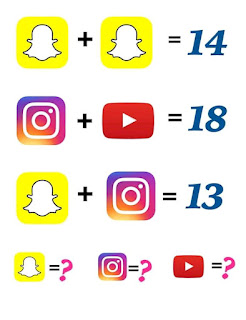## The cat egg and banana puzzle answer

This Social Media puzzle is Trending online and many people failed it. Today I will solve it in detail and give you the correct answer.

## Here's the social media puzzle:

If Snapchat + Snapchat = 14

Snapchat +Instagram = 13

Then Snapchat = ? Instagram = ? And youtube = ?.

See picture belowSnapchat Instagram YouTube puzzle answer

I will solve each equation and substitute in others to get the correct answer.

Equation one: Snapchat+ Snapchat = 14

Implies Snapchat = 7

I will first go to equation three to get the value of Instagram since I already have Snapchat.

Equation three

Snapchat + Instagram = 13

7 + Instagram = 13

Instagram = 13-7 = 6.

Equation two

Hence, Snapchat = 7, Instagram = 6  and YouTube= 13